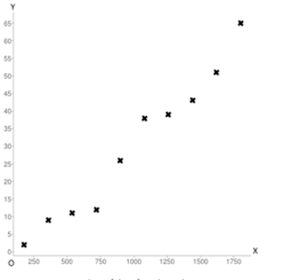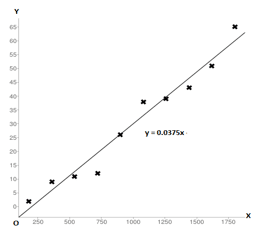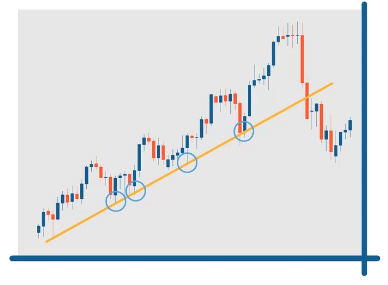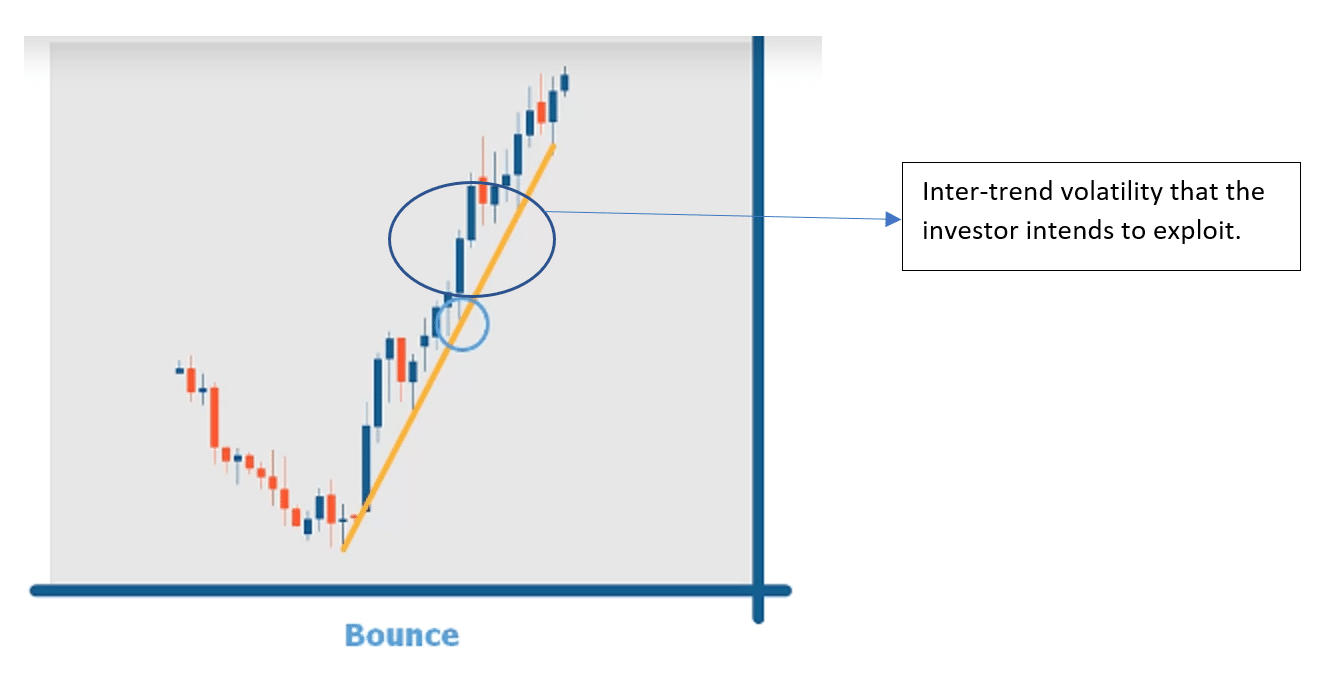Since 2013

• 100% Quantified, data-driven and Backtested
• We always show our results!
• Signals every day via our site or email
• Cancel at any time!

# How to draw a trend line

Last Updated on 20 April, 2023 by Samuelsson

Trend lines are great tools for visual traders and can be used to both gauge the trend direction and find zones where the price is more likely to bounce.

You draw a trend line by connecting two significant higher highs, or two lower lows with a line. The trend line may act as support or resistance, depending on whether the market is trading above or below the trend line. In a bullish trend, the trend lines are rising, unlike in a bearish trend, where they’re falling.

Still, there are many other types of trendlines beyond the most simple one. Below, you will learn a number of other ways of calculating and drawing a trend line.

## How do I calculate trend line?

### Calculating the trend line in three easy steps.

Calculating linear trend lines requires the following three simple steps.

Step 1: draw a scatter plot of the price of an asset.

Scatter plots are created by taking the price of an asset on the y-axis and the time value on the y-axis.

Note: the time values can be in any time-frame such as hours, days, weeks or even years.

Once you have calculated your scatter plot, it will look something like the following figure.Step2: draw a line that has roughly equal number of points above and below the line.This line is your trend line. This trend line is a representation of the general relationship between time and the price of an asset.

Note: this line originates form the origin but not all trend lines originate form the origin.

Step 3: find the equation of a line.

Once the trend line has been drawn, it is a simple matter of basic math to calculate the equation for that trend line.

Any linear trend line will be in the form of

y = mx + c.

y (the price of an asset) and x (a given time period) are variables.

An investor will have to calculate the value of m and c.

Step 3.1: realize m is the slope of the line and use the following formulae calculate the slope of the line

M =

Rise = y2 – y1

Rune = x2 – x1

In simple terms, it’s a difference of prices divided by a difference between different time periods.

For the above diagram and the value of m (slope) comes out to be 0.0375

Step 3.2: calculate the value of c by realizing that c is the y intercept which is the point where the trend line intersects the y-axis.

Since the line originates from the origin, the y-intercept in this case will be zero.

Step 3.3: write the equation in the form of y = mx + c.

Now that the values for m and c have been calculated. One needs to write it in the equation form:

Y = 0.0375x + 0.

Now that the calculation of trend line has been explained, the discussion can move to its relevance for trading.

## Do I need to know how to calculate the trend line?

An investor needs to know the working behind trend lines in order to understand how to use it. In the real world, software will calculate the trend line and even recommend the type of trend line to use for a given data set. Understanding comes in handy when an investor wants to apply a trading strategy on market data. Sometimes an investor will be able to trust the trend line while at the other times they will have to ignore it. Knowing when to ignore and when to trust the trend line is not possible without understanding the calculation for that trend line. Now before one can move towards strategic use of trend lines it is important to gloss-over the main types of trend lines.

## What are the different types of trend lines?

For a statistician there an umpteenth number of ways to categorize the different types of trend lines. However, an investor is only interested in three broad categories.

• Linear trend
• Non-linear trends
• Logarithmic
• Polynomial
• Power
• Exponential
• Moving average trend

The following section will cover the usage of linear trend. Non-linear trends are not in common usage with most investors. However, moving average trend is widely used in a number of trading strategies. For now, the focus is on using the understanding of linear trends to maximize trading returns.

## How do we use the trend line to maximize our profits?

Trend lines are a good tool to first detect the presence of a trend. Then, they can also be used to time one’s entry and exit into the market using various strategies. For starters, investors need to be familiar with the basics of trend lines and their role in detecting trends.

### Using trend lines to detect whether the market is in an uptrend or a down trend.

An investor can make good money by buying when the price for their asset is going up and selling as soon as the price for their asset goes down. This would have been really simple if prices moved in one direction, either up or down. However, reality is far more complicated. Prices go up and down all the time. The movement is random that economists term the movement of price as “random walk”. The following image shows an example of this random movement.Note: In hindsight, these patterns are very clear and can be judged pretty fairly. However, an investor cannot tell that the market will enter a big rally on the 400th day.

The mercurial nature can become a real hassle for investors looking to make a good buck on the market. Luckily, there is a method to the madness of the price movement. Generally, the price is either moving in an uptrend or a downtrend. Please look at the image below for an example.The price is moving randomly for the untrained eye. Notice that the candles have blue color as well as red color. However, after applying the orange colored trend line, the investor will be able to realize that the markets have two distinct trends. Initially, the market was in a down trend and after a reversal the market turned into an uptrend.

Now Detection of up-trends and down trends lies at the core of strategies that use trend lines. Before moving towards strategies of using trend lines, it is important to understand that not all points on the price graph need to be placed directly on the trend line. As a rule of thumb, just connecting two of the lows is enough to draw a line as shown in the following image.Furthermore, it is also not important to have all the price values above or below a trend line. If most of the price values are above or below a trend line, it is more than sufficient. For example, the image below is an example of a valid trend line even though some of the prices are below the trend line.Now one can move towards trading strategies using trend lines.

## What strategies can an investor implement using trend lines?

There are many strategies to use with the trend line. Below, the two most useful strategies will be explained in detail. The first strategy is called a bounce strategy. A bounce strategy is used to make profits within a trend. Afterwards this section will discuss the break strategy which can be used to discuss a change in the trend.

Related reading: Linear vs. Logarithmic Chart

### Bounce strategy of using a trend line

In the bouncing strategy of a trend line, the investor will buy the stock when the price touches the trend line. Doing so would maximize the returns for the investor because they will be exploiting intra-trend variability within the price. Needless to say, the bouncing strategy works best in a market with a lot of variability. The following image shows a perfect situation for using a bouncing strategy.It is important to note that the stop-loss order will play an important role in this strategy in case the investor wants to automate the process. After entering the market at the trend line, the investor can place a stop-loss on the opposite end of the trend-line in order to secure their gains within inter-trend volatility.

### Detecting a break in the trend

An investor can use trend line as a litmus test of whether a trend has ceased to exist or not. If the price value consistently comes below the trend line value, then the one can conclude that the trend has indeed ended. The following image shows a scenario where the break test is used to determine whether the trend has ended or not.## Conclusion

In summary, trend-lines are simple tools that can help an investor make sense of the market and in the process maximize their returns.